# 基于支持向量机的机械振动信号识别与分析Recognition and Analysis of Mechanical Vibration Signal Based on Support Vector Machine

DOI: 10.12677/JISP.2019.83020, PDF, HTML, XML, 下载: 428  浏览: 1,725

Abstract: With the continuous development of modern science and technology and industrialization, me-chanical equipment has become more and more complex, and the degree of integration and auto-mation has been constantly improved, and the links between components have become more closely. Therefore, when the equipment is in operation, if a small part of the fault occurs, these changes will cause the paralysis of the entire equipment, which will not only cause huge economic losses, but also may cause casualties. If we can make use of the health diagnosis technology of ma-chinery and equipment and understand its health status as soon as possible, we can reduce the in-cidence of accidents, so that enterprises can obtain greater benefits. In this paper, we collect signals from different mechanical vibration states, extract the frequency domain characteristics of the signals, and make principal component analysis of the characteristics. Finally, we use support vector machine to recognize various behavior signals, so as to realize the fast and intelligent anal-ysis of vibration signals. The experimental results show that four channels (CH1 - CH4) and six kinds of vibration signals can be basically distinguished by extracting frequency domain features and machine learning algorithm.

1. 引言

2. 振动信号目标特征提取及识别算法

2.1. Fourier分析

$F\left(\omega \right)={\int }_{-\infty }^{+\infty }x\left(t\right){e}^{-i\omega t}\text{d}t$ (1)

$x\left(t\right)=\frac{1}{2\text{π}}{\int }_{-\infty }^{+\infty }F\left(\omega \right){e}^{i\omega t}\text{d}\omega$ (2)

2.2. 小波分析

${\psi }_{a\tau }\left(t\right)=\frac{1}{\sqrt{a}}\psi \left(\frac{t-\tau }{a}\right)$ (3)

$x\left(t\right)\in {L}^{2}\left(R\right)$ 是平方可积函数，ψ(t)是基小波或“母小波函数”，则

$W{T}_{x}\left(a,\tau \right)=\frac{1}{\sqrt{a}}\int x\left(t\right){\psi }^{*}\left(\frac{t-\tau }{a}\right)\text{d}t$ (4)Figure 1. Wavelet function

2.3. 主成分分析

$\stackrel{^}{w}=\underset{{‖w‖}^{2}=1}{\mathrm{arg}\mathrm{min}}{w}^{T}{X}^{T}Xw$ (5)

2.4. 支持向量机

$w\ast x+b=0$ (6)

${y}_{i}\left[w\ast {x}_{i}+b\right]\ge 1-{\xi }_{i},\text{\hspace{0.17em}}i=1,2...,n,\text{\hspace{0.17em}}{\xi }_{i}\ge 0$ (7)

$\stackrel{^}{w}=\mathrm{arg}\underset{w}{\mathrm{min}}\frac{1}{2}{‖w‖}^{2}+C\underset{i=1}{\overset{n}{\sum }}{\xi }_{i}$ (8)

$f\left(x\right)=sign\left(\underset{i=1}{\overset{n}{\sum }}{y}_{i}{\alpha }_{i}^{\ast }\left({x}_{i}\ast x\right)+{b}^{\ast }\right)$ (9)

3. 振动信号目标识别实验

3.1. 样本说明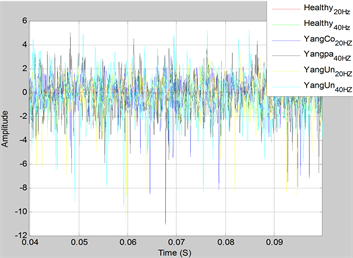Figure 2. CH1 six kinds of time domain samples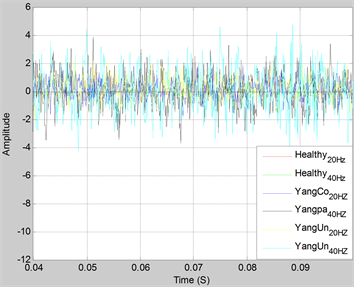Figure 3. CH2 six kinds of time domain samples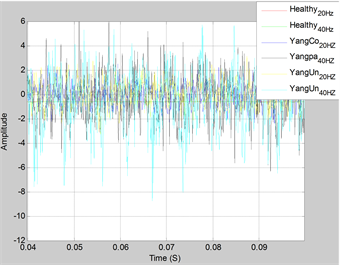Figure 4. CH3 six kinds of time domain samples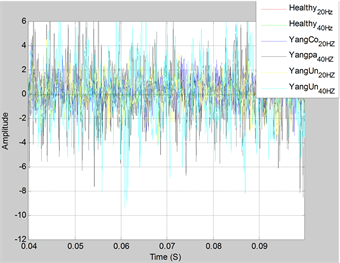Figure 5. CH4 six kinds of time domain samplesFigure 6. Healthy20Hz CWT chart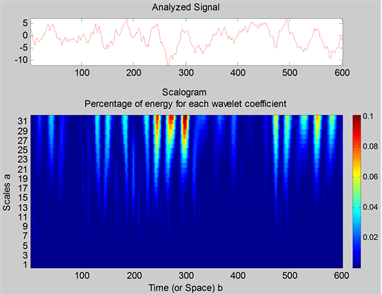Figure 7. Healthy40Hz CWT chart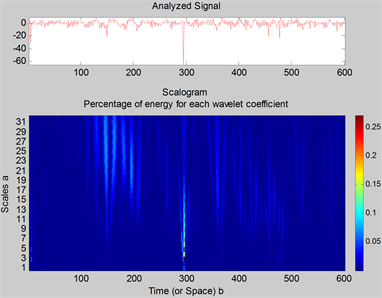Figure 8. YangCo20HZ CWT chart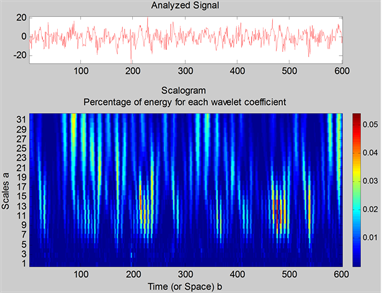Figure 9. Yangpa40HZ CWT chart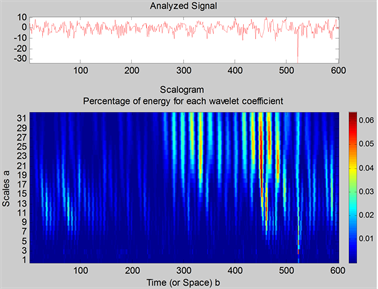Figure 10. YangUn20HZ CWT chart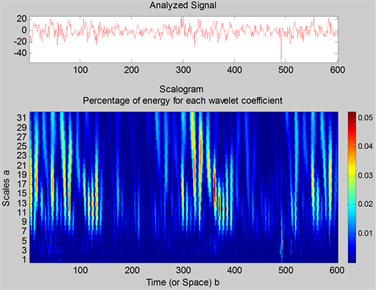Figure 11. YangUn40HZ CWT chart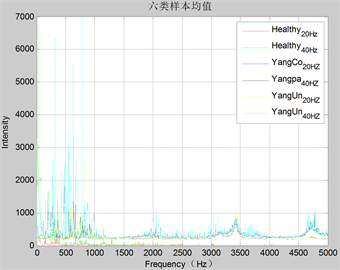Figure 12. FFT means of six kinds of CH1 samplesFigure 13. FFT means of six kinds of CH2 samples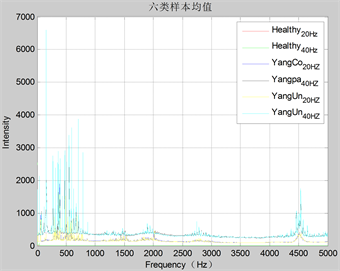Figure 14. FFT means of six kinds of CH3 samples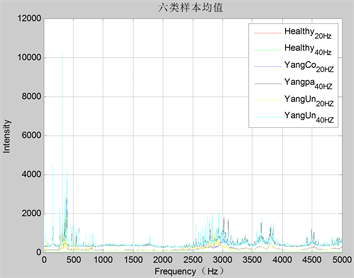Figure 15. FFT means of six kinds of CH4 samplesTable 2. Feature extraction and classifier description

3.2. 实验结果分析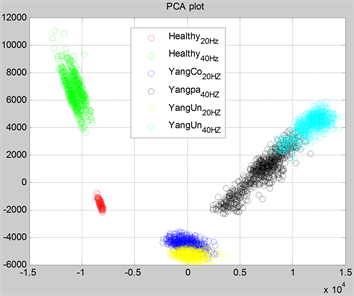Figure 16. FFT feature of six kinds of CH1 samples for PCA chart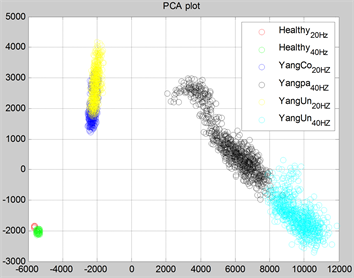Figure 17. FFT feature of six kinds of CH2 samples for PCA chart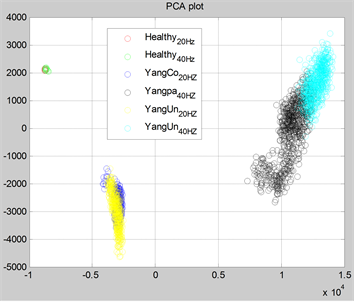Figure 18. FFT feature of six kinds of CH3 samples for PCA chart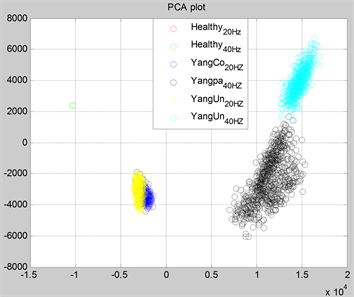Figure 19. FFT feature of six kinds of CH4 samples for PCA chart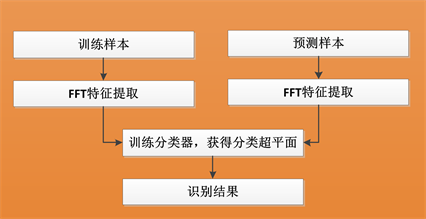Figure 20. Flow of supervised pattern recognition

10次平均结果，测试总体分类正确率及每类样本分类正确率如表3所示：Table 3. Comparison of classification results

4. 总结

  盛兆顺. 设备状态监测与故障诊断技术及应用[M]. 北京: 化学工业出版社, 2003.  陈仲生, 杨拥民, 沈国际. 独立分量分析在直升机齿轮箱故障早期诊断中的应用[J]. 机械科学与技术, 2004, 23(4): 481-483+500.  李强, 王太勇, 胥永刚. 基于混沌和二维近似熵的滚动轴承故障诊断[J]. 振动工程学报, 2007, 20(3): 268-273.  王燕花. 新型光纤传感系统的研究与实现[D]: [博士学位论文]. 北京: 北京交通大学, 2009.  杨渤, 程化, 陈树琪, 等. 基于傅里叶分析的超表面多维光场调控(特邀综述)[J]. 光学学报, 2019, 39(1): 1-20.  Mallat, S.G. and Zhang, Z. (1993) Matching Pursuits with Time-Frequency Dic-tionaries. IEEE Trans on Signal Processing, 41, 3397-3415. https://doi.org/10.1109/78.258082  虞和济, 陈长征, 张省. 基于神经网络的智能诊断[J]. 振动工程学报, 2000, 13(2): 202-209.  Tokuda, K., Yoshimura, T., Masuko, T. and Kobayashi, T. (2000) Speech Parameter Generation Algorithms for HMM-Based Speech Synthesis. 2000 IEEE International Conference on Acoustics, Speech, and Signal Processing, Istanbul, Turkey, 5-9 June 2000, 1315-1318.  张鹏. 基于主成分分析的综合评价研究[D]: [硕士学位论文]. 南京: 南京理工大学, 2004.  丁世飞, 齐丙娟, 谭红艳. 支持向量机理论与算法研究综述[J]. 电子科技大学学报, 2011, 40(1): 2-10.  范永东. 模型选择中的交叉验证方法综述[D]: [硕士学位论文]. 太原: 山西大学, 2013.